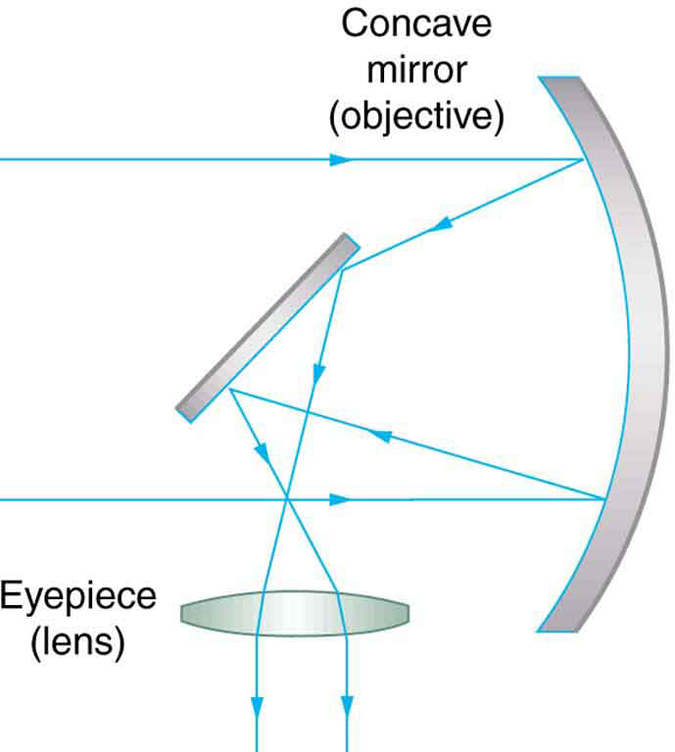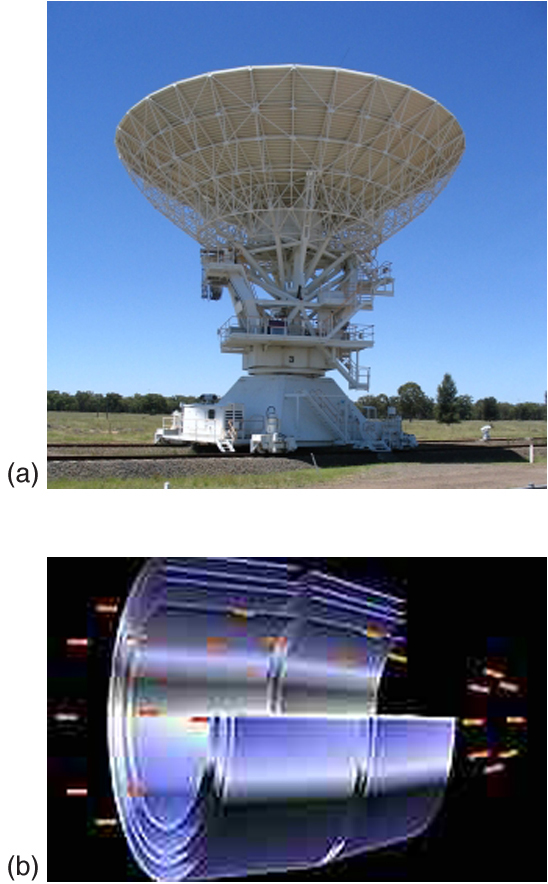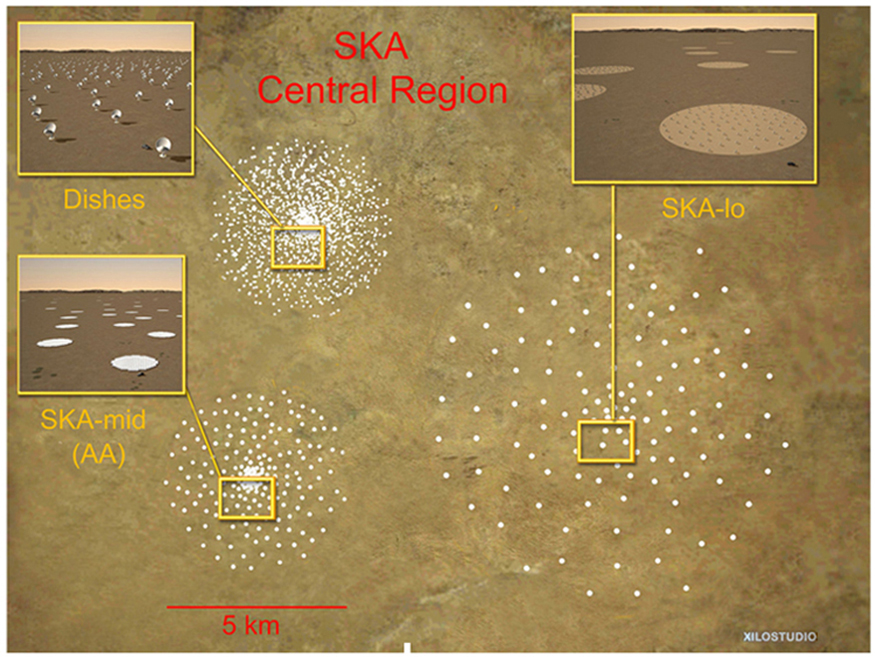# 26.5 Telescopes  (Page 2/4)

 Page 2 / 4

The image in most telescopes is inverted, which is unimportant for observing the stars but a real problem for other applications, such as telescopes on ships or telescopic gun sights. If an upright image is needed, Galileo’s arrangement in [link] (a) can be used. But a more common arrangement is to use a third convex lens as an eyepiece, increasing the distance between the first two and inverting the image once again as seen in [link] .This arrangement of three lenses in a telescope produces an upright final image. The first two lenses are far enough apart that the second lens inverts the image of the first one more time. The third lens acts as a magnifier and keeps the image upright and in a location that is easy to view.

A telescope can also be made with a concave mirror as its first element or objective, since a concave mirror acts like a convex lens as seen in [link] . Flat mirrors are often employed in optical instruments to make them more compact or to send light to cameras and other sensing devices. There are many advantages to using mirrors rather than lenses for telescope objectives. Mirrors can be constructed much larger than lenses and can, thus, gather large amounts of light, as needed to view distant galaxies, for example. Large and relatively flat mirrors have very long focal lengths, so that great angular magnification is possible.A two-element telescope composed of a mirror as the objective and a lens for the eyepiece is shown. This telescope forms an image in the same manner as the two-convex-lens telescope already discussed, but it does not suffer from chromatic aberrations. Such telescopes can gather more light, since larger mirrors than lenses can be constructed.

Telescopes, like microscopes, can utilize a range of frequencies from the electromagnetic spectrum. [link] (a) shows the Australia Telescope Compact Array, which uses six 22-m antennas for mapping the southern skies using radio waves. [link] (b) shows the focusing of x rays on the Chandra X-ray Observatory—a satellite orbiting earth since 1999 and looking at high temperature events as exploding stars, quasars, and black holes. X rays, with much more energy and shorter wavelengths than RF and light, are mainly absorbed and not reflected when incident perpendicular to the medium. But they can be reflected when incident at small glancing angles, much like a rock will skip on a lake if thrown at a small angle. The mirrors for the Chandra consist of a long barrelled pathway and 4 pairs of mirrors to focus the rays at a point 10 meters away from the entrance. The mirrors are extremely smooth and consist of a glass ceramic base with a thin coating of metal (iridium). Four pairs of precision manufactured mirrors are exquisitely shaped and aligned so that x rays ricochet off the mirrors like bullets off a wall, focusing on a spot.(a) The Australia Telescope Compact Array at Narrabri (500 km NW of Sydney). (credit: Ian Bailey) (b) The focusing of x rays on the Chandra Observatory, a satellite orbiting earth. X rays ricochet off 4 pairs of mirrors forming a barrelled pathway leading to the focus point. (credit: NASA)

A current exciting development is a collaborative effort involving 17 countries to construct a Square Kilometre Array (SKA) of telescopes capable of covering from 80 MHz to 2 GHz. The initial stage of the project is the construction of the Australian Square Kilometre Array Pathfinder in Western Australia (see [link] ). The project will use cutting-edge technologies such as adaptive optics    in which the lens or mirror is constructed from lots of carefully aligned tiny lenses and mirrors that can be manipulated using computers. A range of rapidly changing distortions can be minimized by deforming or tilting the tiny lenses and mirrors. The use of adaptive optics in vision correction is a current area of research.An artist’s impression of the Australian Square Kilometre Array Pathfinder in Western Australia is displayed. (credit: SPDO, XILOSTUDIOS)

## Section summary

• Simple telescopes can be made with two lenses. They are used for viewing objects at large distances and utilize the entire range of the electromagnetic spectrum.
• The angular magnification M for a telescope is given by
$M=\frac{\theta \prime }{\theta }=-\frac{{f}_{\text{o}}}{{f}_{\text{e}}}\text{,}$
where $\theta$ is the angle subtended by an object viewed by the unaided eye, $\theta \prime$ is the angle subtended by a magnified image, and ${f}_{\text{o}}$ and ${f}_{\text{e}}$ are the focal lengths of the objective and the eyepiece.

## Conceptual questions

If you want your microscope or telescope to project a real image onto a screen, how would you change the placement of the eyepiece relative to the objective?

## Problem exercises

Unless otherwise stated, the lens-to-retina distance is 2.00 cm.

What is the angular magnification of a telescope that has a 100 cm focal length objective and a 2.50 cm focal length eyepiece?

$-\text{40}\text{.}0$

Find the distance between the objective and eyepiece lenses in the telescope in the above problem needed to produce a final image very far from the observer, where vision is most relaxed. Note that a telescope is normally used to view very distant objects.

A large reflecting telescope has an objective mirror with a $\text{10}\text{.}0 m$ radius of curvature. What angular magnification does it produce when a $3\text{.}\text{00 m}$ focal length eyepiece is used?

$-1\text{.}\text{67}$

A small telescope has a concave mirror with a 2.00 m radius of curvature for its objective. Its eyepiece is a 4.00 cm focal length lens. (a) What is the telescope’s angular magnification? (b) What angle is subtended by a 25,000 km diameter sunspot? (c) What is the angle of its telescopic image?

A $7.5×$ binocular produces an angular magnification of $-7\text{.}\text{50}$ , acting like a telescope. (Mirrors are used to make the image upright.) If the binoculars have objective lenses with a 75.0 cm focal length, what is the focal length of the eyepiece lenses?

$+\text{10.0 cm}$

Consider a telescope of the type used by Galileo, having a convex objective and a concave eyepiece as illustrated in [link] (a). Construct a problem in which you calculate the location and size of the image produced. Among the things to be considered are the focal lengths of the lenses and their relative placements as well as the size and location of the object. Verify that the angular magnification is greater than one. That is, the angle subtended at the eye by the image is greater than the angle subtended by the object.

write an expression for a plane progressive wave moving from left to right along x axis and having amplitude 0.02m, frequency of 650Hz and speed if 680ms-¹
how does a model differ from a theory
what is vector quantity
Vector quality have both direction and magnitude, such as Force, displacement, acceleration and etc.
Besmellah
Is the force attractive or repulsive between the hot and neutral lines hung from power poles? Why?
what's electromagnetic induction
electromagnetic induction is a process in which conductor is put in a particular position and magnetic field keeps varying.
Lukman
wow great
Salaudeen
what is mutual induction?
je
mutual induction can be define as the current flowing in one coil that induces a voltage in an adjacent coil.
Johnson
how to undergo polarization
show that a particle moving under the influence of an attractive force mu/y³ towards the axis x. show that if it be projected from the point (0,k) with the component velocities U and V parallel to the axis of x and y, it will not strike the axis of x unless u>v²k² and distance uk²/√u-vk as origin
show that a particle moving under the influence of an attractive force mu/y^3 towards the axis x. show that if it be projected from the point (0,k) with the component velocities U and V parallel to the axis of x and y, it will not strike the axis of x unless u>v^2k^2 and distance uk^2/√u-k as origin
No idea.... Are you even sure this question exist?
Mavis
I can't even understand the question
yes it was an assignment question "^"represent raise to power pls
Gabriel
Gabriel
An engineer builds two simple pendula. Both are suspended from small wires secured to the ceiling of a room. Each pendulum hovers 2 cm above the floor. Pendulum 1 has a bob with a mass of 10kg . Pendulum 2 has a bob with a mass of 100 kg . Describe how the motion of the pendula will differ if the bobs are both displaced by 12º .
no ideas
Augstine
if u at an angle of 12 degrees their period will be same so as their velocity, that means they both move simultaneously since both both hovers at same length meaning they have the same length
Modern cars are made of materials that make them collapsible upon collision. Explain using physics concept (Force and impulse), how these car designs help with the safety of passengers.
calculate the force due to surface tension required to support a column liquid in a capillary tube 5mm. If the capillary tube is dipped into a beaker of water
find the time required for a train Half a Kilometre long to cross a bridge almost kilometre long racing at 100km/h
method of polarization
Ajayi
What is atomic number?
The number of protons in the nucleus of an atom
Deborah
type of thermodynamics
oxygen gas contained in a ccylinder of volume has a temp of 300k and pressure 2.5×10Nm
why the satellite does not drop to the earth explain
what is a matter
Yinka
what is matter
Yinka
what is matter
Yinka
what is a matter
Yinka
I want the nuclear physics conversation
Mohamed
because space is a vacuum and anything outside the earth 🌎 can not come back without an act of force applied to it to leave the vacuum and fall down to the earth with a maximum force length of 30kcm per second
Clara

#### Get Jobilize Job Search Mobile App in your pocket Now!ByBy OpenStaxBy Madison ChristianBy DanielrosenbergerBy OpenStaxBy Donyea SweetsBy David BourgeoisBy OpenStaxBy Yasser IbrahimBy OpenStaxBy Alec Moffit